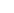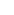# Maths Resources (page 4)Browse ResourcesTutor Hunt Resources### Fraction and it`s types

It represents the equal parts of the whole. A fraction has two parts, namely numerator and denominator. The number on the top is called the numerator, and the number on the bottom is ...

### What is a power? What are indices?

A power is another word for indices (what we looked at briefly during the BIDMAS section). Powers frequently pop up in mathematics, and to recognise them, it is usually a small number...

### How to Use Language to Solve Maths Problems

This is a research paper by &Neil Mercer Faculty of Education, University of Cambridge, UK and Claire Sams Faculty of Education and Language Studies, The Open University, Milton Ke...

### Using/Drawing Pie Charts

Pie Charts are used to quickly represent the Proportions of each Group of Data.We do this by taking `Slices` of a Circle, that are proportional towards the size of each group. For ins...

### What is meant exactly by `BIDMAS`?

`BIDMAS` or `BODMAS` is a term we hear Maths Teachers use often, but what does it actually mean? Here`s a quick and easy explanation.Let`s take a look at the following calculation:7 x...### What is hypothesis testion?

Hypothesis testing is an act in statistics whereby an analyst tests an assumption regarding a population parameter. The methodology employed by the analyst depends on the nature of th...

### time trap!

A time trap is a saying I introduce to my students to help them identify a question that could have a negative impact on their ability to perform in the exam. Perhaps the examiner has...

### Prime Numbers

A prime number is simply a number that is only divisible by itself and the number 1. Therefore, it has only two factors.For example, is the number 7 a prime number? To figure this out...

### How to solve simple linear equations

Simple linear equations can appear on GCSE examination papers across all three tiers, so are an absolute must for understanding.Linear equations tend to be of the form:6x + 5 = 10 or ...

### Arithmetic Sequences

An arithmetic sequence, also known as an linear sequence, is a series of numbers where the difference between the numbers remains constant. Pupils may be asked to work out the nth ter...
See All ResourcesView All Subjects